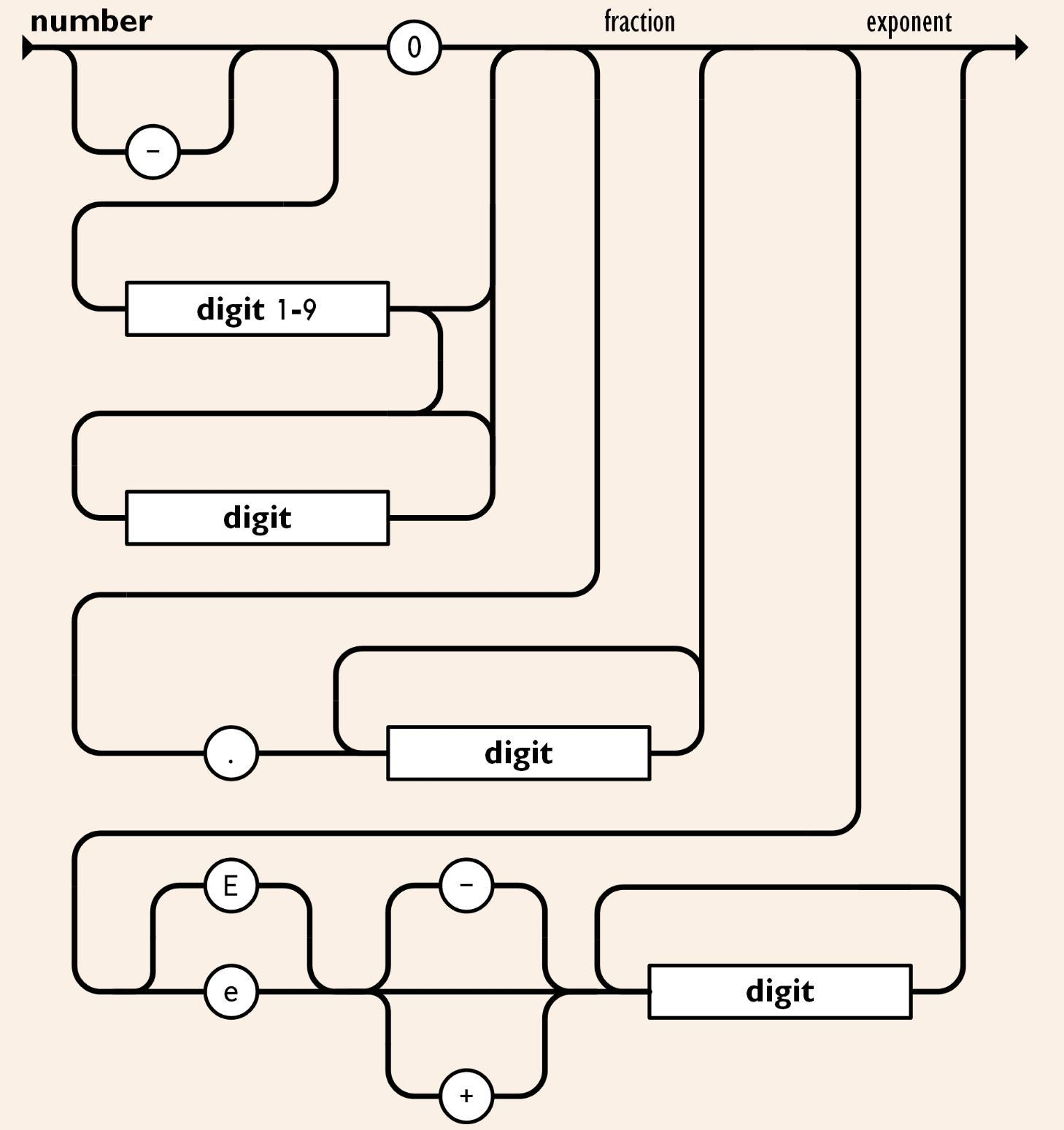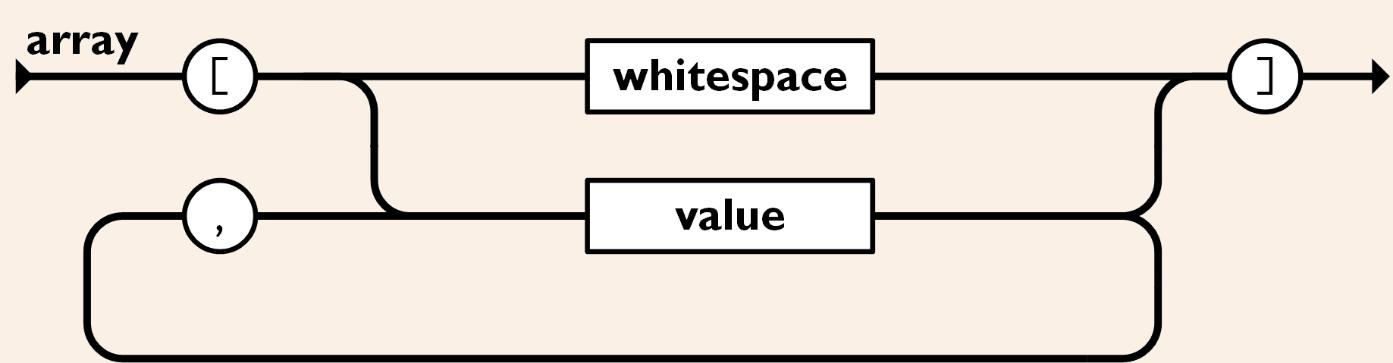# 從無到有寫一個 JSON 解析器（2）

part1 當中我們提到了如何撰寫一個 JSON 解析器，並實作解析字串的功能，接下來我們把其他函數補上。（事實上只要知道基本原理，剩下的函數實作都是照本宣科而已）

## Numbernumber 實作上也不難，容易忽略的地方在於小數點、負數、浮點數的部分，還有指數表達（1e6）。（話說剛剛才發現 E 也可以）

javascriptfunction number(parser) {  let str = "";  if (parser.current() === "-") {    str += "-";    parser.index += 1;  }  let curr = "";  while (((curr = parser.current()), curr >= "0" && curr <= "9")) {    str += curr;    parser.index += 1;  }  let isFloat = false;  // float number  if (parser.next(".")) {    str += ".";    isFloat = true;    while (((curr = parser.current()), curr >= "0" && curr <= "9")) {      str += curr;      parser.index += 1;    }  }  // exponential expression  let expo = "";  if (parser.next("e")) {    curr = "";    if (parser.next("-")) {      expo += "-";    }    while (((curr = parser.current()), curr >= "0" && curr <= "9")) {      expo += curr;      parser.index += 1;    }  }  if (expo) {    return isFloat      ? parseFloat(str, 10) * Math.pow(10, +expo)      : parseInt(str, 10) * Math.pow(10, +expo);  }  return isFloat ? parseFloat(str, 10) : parseInt(str, 10);}
• 第一個部分，我們先看看它是不是負數
• 第二部分，跑 while 迴圈把數字部分放入
• 第三部分，判斷是否有小數點

• 如果有的話就再爬一次數字
• 第四部分，判斷是否有指數表達（大寫或小寫 e）

• 如果有的話也再爬一次數字
• 第五部分：把字串變成數字（使用 parseInt 或是 parseFloat）

## 關鍵字（true, false, null）

javascriptfunction keyword(parser) {  if (parser.next("true")) {    return true;  } else if (parser.next("false")) {    return false;  } else if (parser.next("null")) {    return null;  }}

## 陣列（Array）javascriptfunction array(parser) {  const arr = [];  if (parser.current() === "[") {    parser.next("[");    parser.skip();    if (parser.next("]")) {      return arr;    }    let i = 0;    while (parser.current()) {      const val = value(parser);      arr.push(val);      parser.skip();      if (parser.current() === "]") {        parser.next("]");        return arr;      }      parser.next(",");      parser.skip();    }  }  return arr;}
• 第一個部分，先看看是否是 [ 開頭

• 如果遇到 ] 代表他是一個空陣列
• 第二部分，跑 while 迴圈執行 value 函數，並放到 array 當中
• 遇到 ] 代表陣列結尾，回傳 array。
• 遇到逗號代表還有下一個元素，繼續執行

javascriptconst escape = {  '"': '"',  t: "\t",  r: "\r",  "\\": "\\",};while (((curr = parser.current()), curr)) {    if (parser.next('"')) {      return str;    } else if (curr === "\\") {      parser.index += 1;      const escapeCh = parser.current();      if (escape[escapeCh]) {        str += escape[escapeCh];      }    } else {      str += curr;    }    parser.index += 1;  }

## 客製功能：$template$

json{  "name": $name$}

javascriptnew Parser(string, { name: 'kalan' }).parse();// { name: "kalan" }

### 實作

javascriptfunction template(parser) {  parser.skip();  if (parser.next("$")) { parser.skip(); if (parser.next("$")) {      throw new Error("template can not be empty");    }    let curr = "";    let key = "";    while (((curr = parser.current()), curr)) {      if (parser.next("$")) { return parser.variables[key]; } key += curr; parser.index += 1; } }} • 首先先匹配 $
• 開始讀取內容，直到看到 $ • 遇到 $ 停止 while 迴圈並用變數將模板變數取代，回傳結果

Buy me a coffee